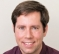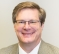## Digital PID controller in velocity formStarted by 4 years ago4 replieslatest reply 4 years ago1669 views

Hello all,

I have been facing a problem how to implement digital PID controller. I have

studie a lot of books and articles about that and in all the books I have read

so far it is mentioned that velocity form of PID controller (which is described

by the below given difference equation) is the most sutaible implementation of

the digital PID controller because of easy antiwind up and bumpless transfer

implementation.

$$\Delta u(k) = \Delta u_p(k) + \Delta u_i(k) + \Delta u_d(k)$$

$$\Delta u_p(k) = u_p(k) - u_p(k-1) = K_p\cdot[e(k) - e(k-1)]$$

$$\Delta u_i(k) = u_i(k) - u_i(k-1) = K_p\cdot T/T_i\cdot[e(k) + e(k-1)]$$

(in case the trapezoidal integration is used)

$$\Delta u_d(k) = u_d(k) - u_d(k-1) = K_p\cdot T_d/T\cdot[e(k) - 2\cdot e(k-1) + e(k-2)]$$

(in case the backward difference is used, filtering pole

has been omitted)

$$u(k) = u(k-1) + \Delta u(k)$$

Unfortunately I haven't found any example how to implement neither antiwind-up

nor bumpless transfer mechanism for the PID controller in velocity form so I have

been attempting to invent that by myself.

Here are my ideas:

a) how to implement antiwind-up

If {([u(k-1) == umax] && [du(k) > 0]) ||

([u(k-1) == umin] && [du(k) < 0])}

then u(k)=u(k-1)

b) how to implement bumpless transfer

In my opinion for bumpless transfer it is sufficient to update the u(k-1) with

value set in manual mode.

Please can anybody tell me whether my ideas are correct? Thank you for any suggestions.

[ - ]Yes, both of your ideas are correct.

That said, I really don't like implementing anti-windup by limiting the integrator state.  I much prefer to have limits on the total controller output that match the downstream actuator limits, and then avoid updating the integrator when the controller as a whole (P + I + D) would exceed the limits.

I had actually never heard of the "velocity form" of the PID controller before this question, despite having been a controls specialist for 15 years.  I personally don't see the benefit, but that may be because I learned it the old-fashioned way, and have trouble looking at it from the beginner's perspective.

[ - ]Interesting approach to anti-windup, I've not seen this before and it makes great sense. If I understand correctly, the idea is to prevent integral state accumulation when there is significant P or D contribution as well as a significant I accumulation. This is consistent with the idea that the purpose of I is to prevent running along with a small but constant error, a case that occurs when very near the set point with small P and D contributions.

It seems worth noting that if the decision is to stick with the originally suggested anti-windup method, it makes sense to use:

u(k-1) >= umax  and:

u(k-1) <= umin

A PID control simulation is always a great approach. That said, modeling PID control is usually straightforward while a modeling a complex plant response can be challenging.

[ - ]I'm not a PID expert. What I would suggest is to write the code && simulate it. run the code in a loop, and "inject" a value, then watch what the code does.

[ - ]